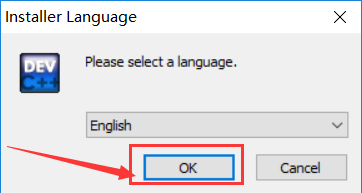# C 代码：

``````//
// Created by NowTime on 2019/2/28.
// 鸡兔同笼 解决程序
//
#include <stdio.h>
#include <stdlib.h>

void main()
{

printf("鸡兔同笼问题解决程序\n");
printf("请务必输入的是正整数！\n");
printf("否则将会卡住...你只能按 Ctrl+C 或 点击右上角 X 关闭本程序\n\n");

printf("请输入“鸡兔数量”：");

printf("请输入“脚数量”：");
scanf_s("%d", &feet);

int rabbit, chicken, count_feet;

count_feet = (chicken*2)+(rabbit*4);//脚的数量 = 鸡的数量*2条腿 +兔子的数量*4条腿

//如果算出脚的数量 等于 输入脚的数量，则输出
if(count_feet == feet){
printf("鸡有：%d只，兔子有：%d\n", chicken, rabbit);
break;//退出循环
}

}

system("pause");//暂停，按任意键退出本程序，需要引入头文件  #include <stdlib.h>

}``````

# 输出结果：

``````PS D:\C语言> ./鸡兔同笼解决程序.exe

# C 代码

``````#include <stdio.h>

void main() {
int i,ii;

for(i=1; i<=9; i++){
for (ii=1;  ii<=i ; ii++) {
printf("%dx%d=%d\t", i, ii, i*ii);
}
printf("\n");
}
}``````

# 运行结果：

``````D:\CLionProjects\hello\cmake-build-debug\hello.exe
1x1=1
2x1=2   2x2=4
3x1=3   3x2=6   3x3=9
4x1=4   4x2=8   4x3=12  4x4=16
5x1=5   5x2=10  5x3=15  5x4=20  5x5=25
6x1=6   6x2=12  6x3=18  6x4=24  6x5=30  6x6=36
7x1=7   7x2=14  7x3=21  7x4=28  7x5=35  7x6=42  7x7=49
8x1=8   8x2=16  8x3=24  8x4=32  8x5=40  8x6=48  8x7=56  8x8=64
9x1=9   9x2=18  9x3=27  9x4=36  9x5=45  9x6=54  9x7=63  9x8=72  9x9=81

Process finished with exit code 0``````

# 代码如下

## 2019-03-11 12:40 更新代码，由于上次仓促，未考虑周全，今天抽空更新一下

``````/**
* 计算当前是本学期的第几周
* 默认是以 2019-02-25 为开学时间
* @param int \$start_timestamp  本学期开始时间戳，可以用 strtotime 函数获取，如 strtotime('2019-02-25')
* @return int
*/
function now_week(\$start_timestamp=1551024000)
{
date_default_timezone_set('Asia/Shanghai');
\$this_week_monday = strtotime("this week Monday", \$start_timestamp);//获取指定时间中，周一时间戳

//使用 ceil 函数进行“向下取整”
// ceil(3.4)    输出结果为 4
// ceil(3.6)    输出结果为 4
return ceil((time()-\$this_week_monday)/604800);
}``````

# 实际应用：# 下载地址

• ## 官方下载地址：https://sourceforge.net/projects/orwelldevcpp/# 1. 安装- 阅读剩余部分 -